Coulombs Law the opposites attract force Coulombs Law

• Slides: 13Coulomb’s Law the “opposites attract” force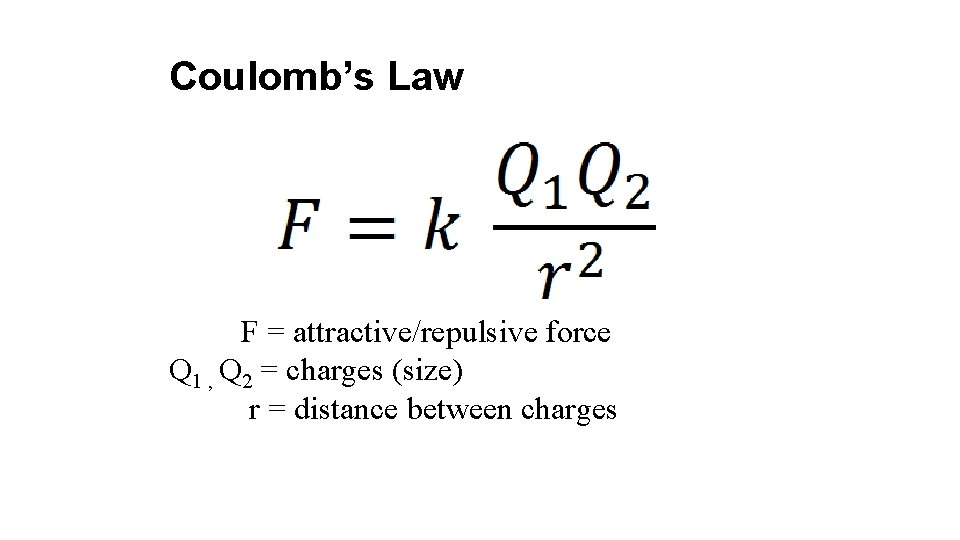Coulomb’s Law F = attractive/repulsive force Q 1 , Q 2 = charges (size) r = distance between charges• Is the force between electrons and the nucleus an attraction or a repulsion?• Is the force between electrons and the nucleus an attraction or a repulsion? It is an attraction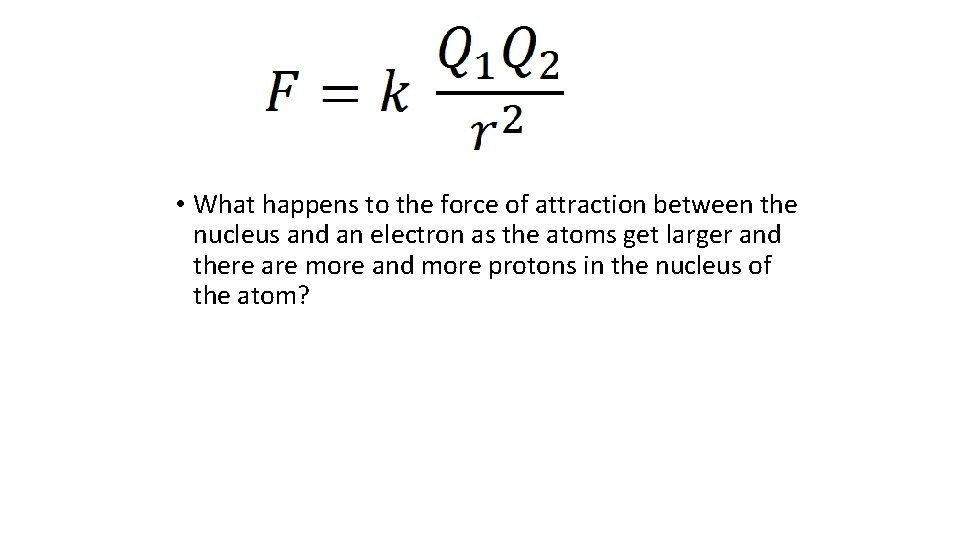• What happens to the force of attraction between the nucleus and an electron as the atoms get larger and there are more and more protons in the nucleus of the atom?• What happens to the force of attraction between the nucleus and an electron as the atoms get larger and there are more and more protons in the nucleus of the atom? • When Q 1 or Q 2 or both get larger, the attractive force increases• What happens to the force of attraction if the distance between the nucleus and the electron gets larger?• What happens to the force of attraction if the distance between the nucleus and the electron gets larger? • As the charges get farther apart the attractive force between them gets smaller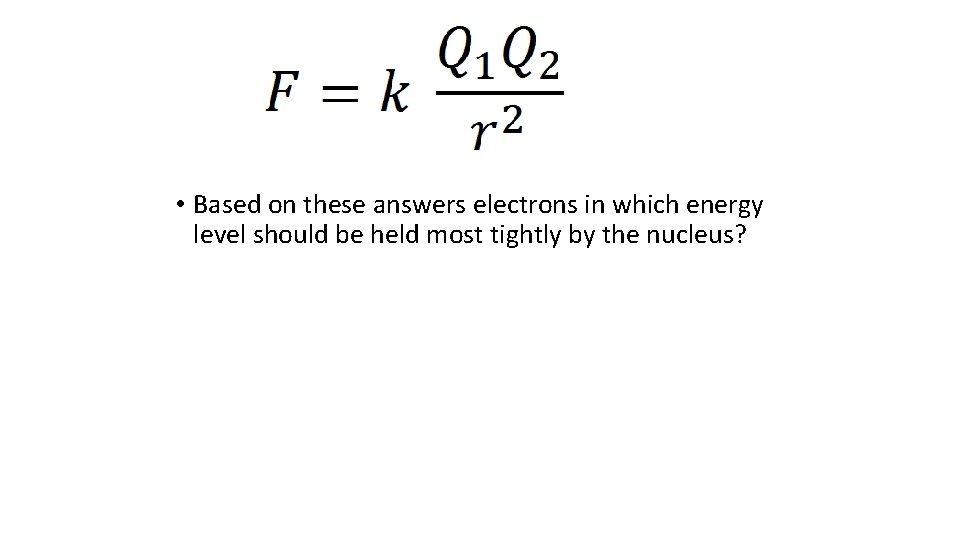• Based on these answers electrons in which energy level should be held most tightly by the nucleus?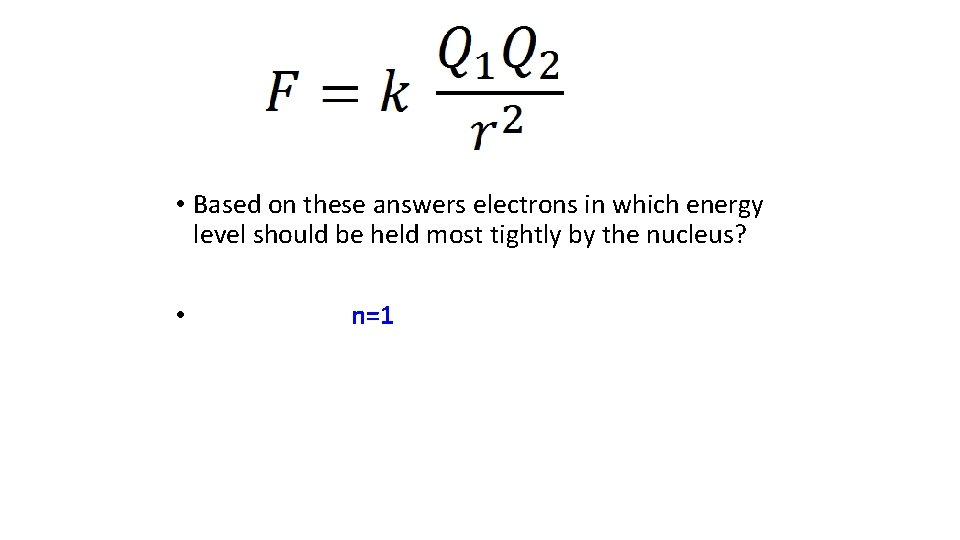• Based on these answers electrons in which energy level should be held most tightly by the nucleus? • n=1• Based on these answers electrons in which orbital within the energy level should be held most tightly by the nucleus? Most loosely?• Based on these answers, electrons in which orbital within the energy level should be held most tightly by the nucleus? Most loosely? • “s” electrons should be held most tightly • Electrons in the last orbital WITHIN AN ENERGY LEVEL being filled should be held most loosely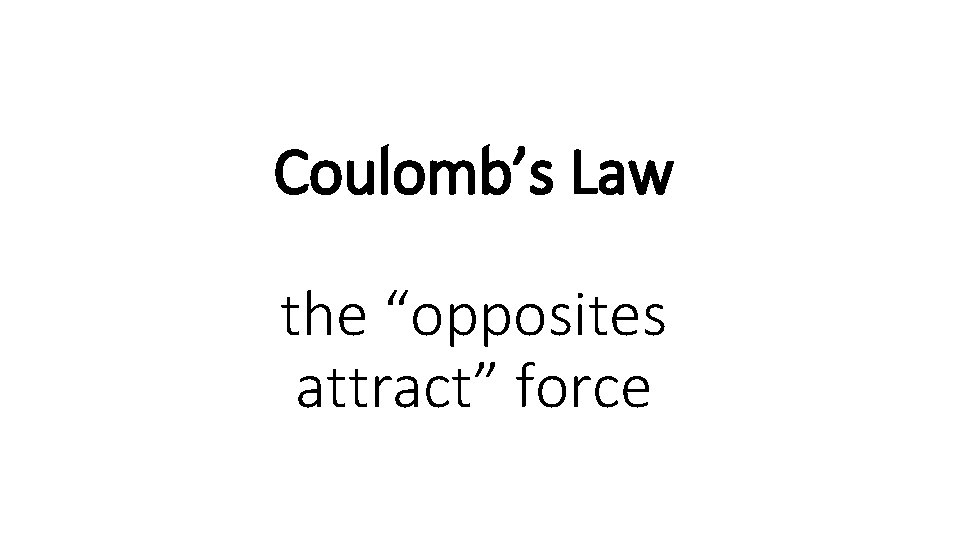Coulomb’s Law the “opposites attract” force